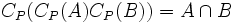# Centralizer-large subgroups permute and their product and intersection are centralizer-large

## Statement

Suppose$P$ is a group of prime power order and$A,B$ are Centralizer-large subgroup (?)s of$P$. Then,$AB = BA$, and both$AB$ and$A \cap B$ are both centralizer-large subgroups of$P$.

## Facts used

1. Product formula

## Proof

Given: A group$P$ of prime power order, centralizer-large subgroups$A,B$ of$P$.

To prove:$AB = BA$, and both$AB$ and$A \cap B$ are also centralizer-large subgroups.

Proof: Note that$AB = BA$ if and only if it is a subgroup, if and only if it is equal to$\langle A, B \rangle$. In general, we have$|AB| \le \left| \langle A,B \rangle \right|$.

By fact (1), we have:

$|A||B|}{|A \cap B|} \tag{(*)$

and:

$|C_P(A)||C_P(B)|}{|C_P(A) \cap C_P(B)|} \tag{(*)$

Also, we have:

$(**)$

and:

$(***)$ .

Denote by$f_1(D)$ the value$|D||C_P(D)|$. Then, combining (*) and (**), we see that:$f_1(A \cap B)f_1(C_P(A) \cap C_P(B)) \ge f_1(A)f_1(B)$

with equality holding in all the inequalities. But$A$ and$B$ maximize$f_1$, hence equality must hold. Thus,$AB = BA = C_P(C_P(A) \cap C_P(B))$,$C_P(B)C_P(A) = C_P(A)C_P(B) = C_P(A \cap B)$. Also,$C_P(AB) = C_P(A) \cap C_P(B)$ and$C_P(C_P(A)C_P(B)) = A \cap B$ by definition. Thus, the subgroups$A \cap B$ and$AB$ are centralizer-large.

Since$f_1$ is maximized for$A$ and$B$, we must have equality. Hence$AB = BA$. Moreover, we get that$C_P(AB) = C_P(A) \cap C_P(B)$ and$C_P(C_P(A)C_P(B)) = A \cap B$.